# Test: Viscosity - 1

## 10 Questions MCQ Test Fluid Mechanics | Test: Viscosity - 1

Description
Attempt Test: Viscosity - 1 | 10 questions in 25 minutes | Mock test for Civil Engineering (CE) preparation | Free important questions MCQ to study Fluid Mechanics for Civil Engineering (CE) Exam | Download free PDF with solutions
QUESTION: 1

### Water flows between two plates of which the upper one is stationary and the lower one is moving with a velocity V. What will be the velocity of the fluid in contact with the upper plate?

Solution:

Explanation: According to the No-Slip condition, the relative velocity between the plate and the fluid in contact with it must be zero. Thus, the velocity of the fluid in contact with the upper plate is 0 and that with the lower plate is V.

QUESTION: 2

### The viscous force ____ the relative motion between the adjacent layers of a fluid in motion.Which one of the flowing fits best in the sentence?

Solution:

Explanation: Viscosity is the internal friction of a fluid in motion. It is the property by the virtue of which the relative motion between two adjacent fluid layers is opposed.

QUESTION: 3

### The viscosity of a fluid in motion is 1 Poise. What will be it’s viscosity (in Poise) when the fluid is at rest?

Solution:

Explanation: Viscosity is the property of a fluid and is constant for a given fluid under given conditions, irrespective of the fact whether the fluid is at rest or in motion.

QUESTION: 4

Which of the following correctly states how the viscosities of a liquid and a gas will change with temperature?

Solution:

Viscosity of a liquid is due to the cohesion between it’s molecules. With the increase in temperature of a liquid, cohesion increases, leading to the rise in viscosity. Viscosity of a gas is due to the momentum transfer between it’s molecules. With the increase in the temperature of a liquid, molecular motion increases, leading to the fall in viscosity.

QUESTION: 5

Which one of the following is not a unit of dynamic viscosity?

Solution:

Explanation: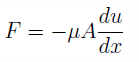where F= viscous force, A= area, du ⁄ dx = velocity gradient, μ = co-effcient of viscosity. Therefore,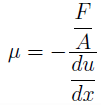SI unit of μ is N-s/m2 = Pa-s and CGS unit of μ is dyne-s/cm2. 1 Poise= 1 dyne-s/cm2 and 1 Stokes= 1 cm2/s. Thus, Stokes is not an unit of μ, rather it is a unit of kinematic viscosity υ.

QUESTION: 6

Newton's law of viscosity states that

Solution: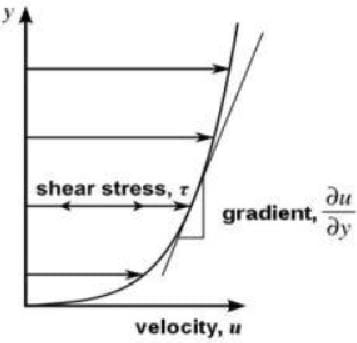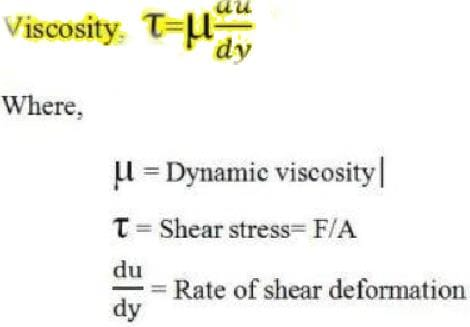QUESTION: 7

Which one of the following is the CGS unit of dynamic viscosity?

Solution:

Explanation: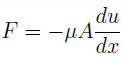where F= viscous force, A= area, du ⁄ dx = velocity gradient, μ = co-effcient of viscosity. Therefore,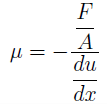CGS unit of μ is = dyne-s/cm2. 1 Poise= 1 dyne-s/cm2 and 1 Stokes= 1 cm2/s. Thus, the CGS unit of μ will be Poise. Stokes is the CGS unit of kinematic viscosity.

QUESTION: 8

The dynamic viscosity of a fluid is 1 Poise. What should one multiply to it to get the answer in N-s/m2

Solution:

Explanation:
1 Poise = 1 dyne-s/cm2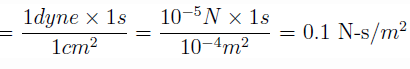QUESTION: 9

Which of the following is a CGS unit of kinematic viscosity?

Solution:
QUESTION: 10

Which of the following is the dimension of kinematic viscosity?

Solution:

Explanation: ν = μ/ρ, where ν = kinematic viscosity, μ = dynamic viscosity and ρ = density of the fluid.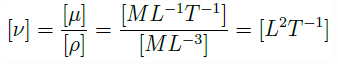Use Code STAYHOME200 and get INR 200 additional OFF Use Coupon Code